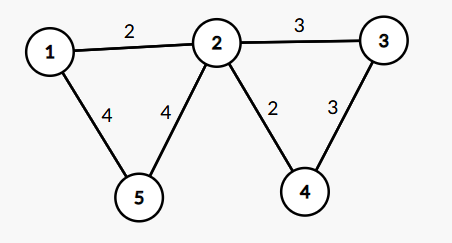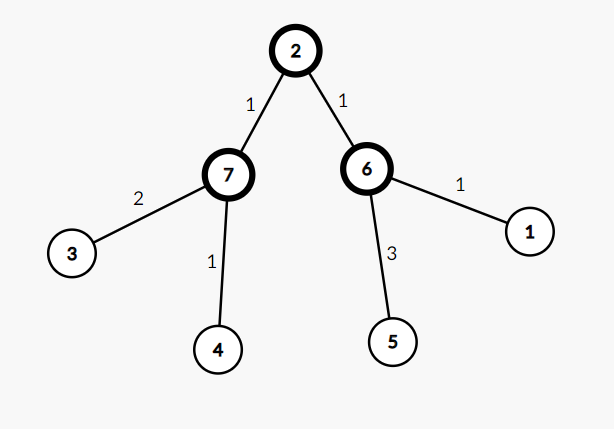P7100 [w3R1] 团

## 题目描述

• 有 $k$ 个集合；第 $i$ 个集合可以表示为 $Si={(T_1,W_1),(T_2,W_2),\dots,(T{|Si|},W{|S_i|})}$。
• 对于任何两对 $(T_i,W_i),(T_j,W_j)$ 在同一个集合里面，图中会形成一条连 $T_i$ 和 $T_j$ 的边，边权为 $W_i+W_j$。

## 题解

1. 暴力建图，跑 Dijkstra，可以通过前 $50\%$ 的数据

2. 对于 $100\%$ 的数据，仍然使用暴力建图并采用优先队列优化的 Dijkstra 就是 $\mathcal{O}(\sum |S^2_i| \log \sum |S^2_i|)$ 的复杂度，不能接受，考虑如何优化建图。

每个集合中所有点总要两两连边，这样边数是 $|S^2| - |S|$($\dfrac{|S| \times (|S|-1)}{2} \times 2$)的，将其转换一下，对于每一个集合设立一个中间点，所有的点 $T_i$ 向其连一条边权为 $W_i$ 的边，和原图实际上是等价的，边数变成了 $2|S|$。

以样例为例：## 注意

1. 本题需要 long long
2. 不一定联通
3. 每一个集合的中间点都是不同的（代码中用 $n+1 \sim n+k$ 表示）